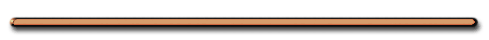HomeTry this at home!

Show this to your young siblings or cousins.
All you need is whole milk, food dye,
and a Qtip soaked dish detergent.

Video Instruction

showmethephysics.com

Instantaneous Velocity - velocity at a single moment in time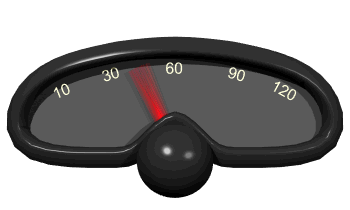What is the velocity of the object
at 6 seconds?

 d (cm)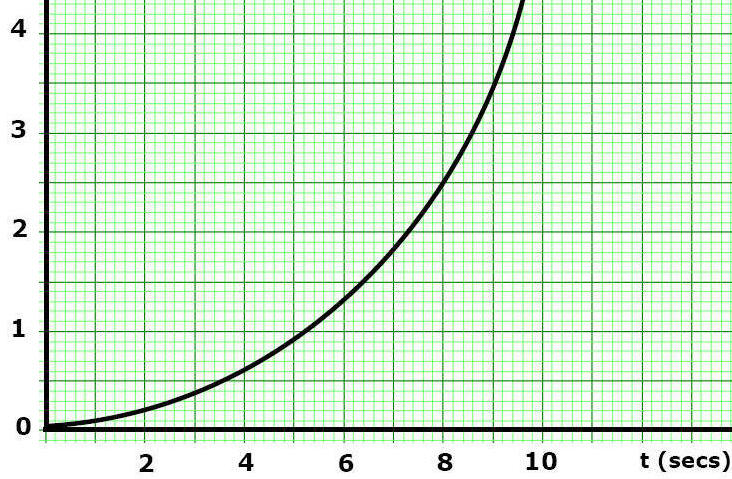Step 1 - Draw a tangent line at the time you want to find the velocity
 d (cm)Step 2 - Find the SLOPE of the tangent line (Slope = Velocity)

 distance (cm)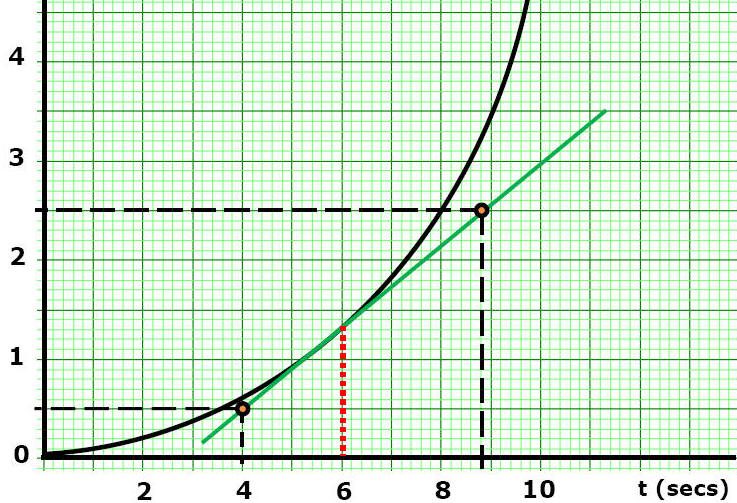Slope of tangent = ∆d/∆t
= Velocity @ 6 sec.

= (2.5 m - .5m)/(8.8 sec - 4 sec)

= .4 m/s

 Rank the accelerations from greatest to least.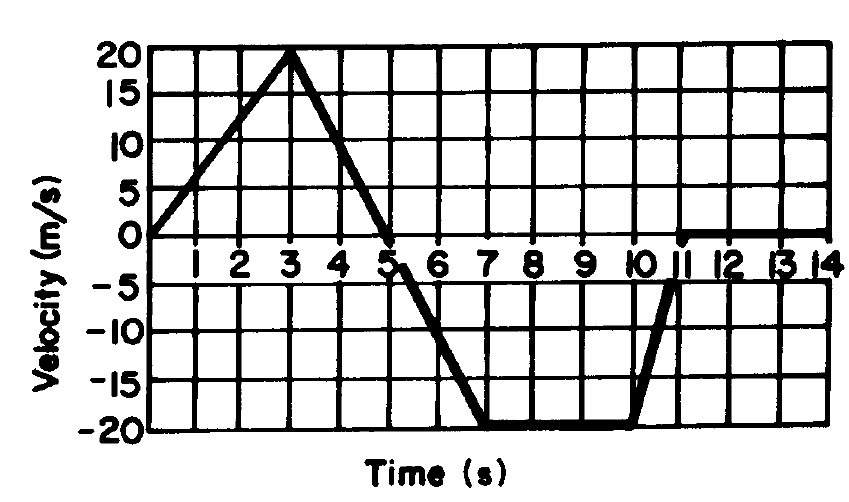Acceleration